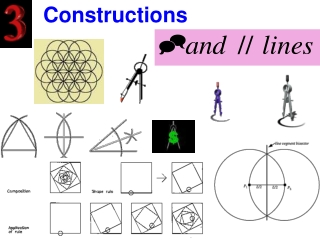Download PresentationConstructions

# Constructions

Download Presentation## Constructions

- - - - - - - - - - - - - - - - - - - - - - - - - - - E N D - - - - - - - - - - - - - - - - - - - - - - - - - - -
##### Presentation Transcript

1. Constructions

2. Find the perpendicular line to L1 through the external point P. Note that the perpendicular bisector of the segment will not go through point P.

3. Do you understand ? We can not use the fish on points A and B.

4. Let us find 2 points on the line that are equidistant from point P. Why ? So we can go fishing. It’s a fluke ! Catfish ?

5. Swing a compass from point P through the segment.

6. This will create a segment cd which has P on its perpendicular bisector. This technique will be called …. The Smile technique

7. Now we construct the fish using points c and d. Swing an arc from point c.

8. Swing an arc from point d. Construct a line through the blue points of intersections.

9. Note that the procedure is just aSmile and a Fish.

10. Let us try this again. Create a perpendicular line though P. Create the smile.

11. Note that the segment needs to be extended. Now, we are ready to construct the fish.

12. Swing arc from point c. Swing arc from point d. Construct line through the points of arc intersections.

13. Review To construct a perpendicular line from an external point to a given line, there are 2 steps. A Smile and A Fish

14. Construct a perpendicular line through point P. Note that the perpendicular bisector of the segment will not go through point P.

15. Look Do you think you know how to construct the perpendicular through P ? Correct ! A smile and a fish.

16. First the smile. Now the fish. Construct an arc from X Construct an arc from y Construct line through the points of arc intersections.

17. Summary Note that the technique for constructing a perpendicular to a line from an exterior point is the same as constructing a perpendicular to a line through a point on the line. A Smile and a Fish.

18. Constructing a // line thru a Pt.

19. Construct a line through P which intersects

20. Duplicate the arc at point P.

21. Set the compass to the distance between the arc intersection points on

22. Swing an arc from bottom point across the top point.

23. Construct // line from the intersection point to point P From above swing an arc from top point across the bottom point.

24. Summary To construct a perpendicular line through either an external or an internal point the technique is called… A Smile and a Fish.

25. Summary To construct a parallel line through an external point, just construct a non-parallel line through the external point. Then duplicate an angle below to the same relative position above.

26. To construct a parallel line to an existing line though an external point 1. Construct a line through both the external point and the existing line. P

27. To construct a parallel line to an existing line though an external point 1. Construct a line through both the external point and the existing line. 2. Copy the new top angle to the right to point P directly above it. P

28. To construct a parallel line to an existing line though an external point 1. Construct a line through both the external point and the existing line. P 2. Copy the new top angle to the right to point P directly above it. 3. Construct the // line through point P and arc intersection point. Voila !

29. C’est fini. Good day and good luck. That’s all folks. A Senior Citizen Production